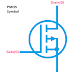The main difference between CMOS and MOSFET is that CMOS is a technology for constructing logical circuits using transistors whereas MOSFET is a device that is used in logic circuits to perform the logical function. CMOS stands for Complimentary Metal Oxide Semiconductor whereas the MOSFET stands for Metal Oxide Semiconductor Field Effect Transistor. So you can understand MOSFET can be used to build a logic circuit using the CMOS technology.

There are two types of MOSFET - P Channel MOSFET(PMOS) and N Channel MOSFET(NMOS). The CMOS is the combination of two PMOS or two NMOS.

A normal MOSFET act as a switch so it can be used for either switching or amplifying. But the CMOS act as an Inverter. The term complementary implies inverting.

First, of all let's know what is a MOSFET. Basically, a MOSFET is a semiconductor device. Its gate terminal is insulated from the channel by a layer of silicon die oxide. That is why it is called a metal oxide semiconductor. A MOSFET has three terminals to connect with an external circuit. The three terminals are - Source, Gate, and Drain.

The charge carrier enters to the channel through the Source terminal and leaves the channel through the Drain terminal.

The gate terminal is used to apply the voltage to the MOSFET to control the current flow. There are two types of MOSFET - enhancement type and depletion type.

In an enhancement type MOSFET, we have to apply a voltage to the MOSFET through its gate terminal to start the current flow. As we increase the voltage the current flow also be increased. On the other hand, the depletion type MOSFET has opposite characteristics. In normal conditions, when there is no voltage to its gate terminal, it will conduct current. To stop or control the flow of current we have to apply a voltage to its gate terminal.

Here, you can see the symbol of P - Channel(PMOS) MOSFET. P - Channel MOSFET required a positive voltage to its gate terminal with respect to the source terminal.

Now, here you can see the symbol of N - Channel MOSFET. N - channel(NMOS) MOSFET requires negative voltage to its gate terminal with respect to the source terminal.

The CMOS circuit is built with both PMOS and NMOS. Here you can see the circuit diagram CMOS using PMOS and NMOS. You can see it is built with MOSFETs.

Now, you can see the symbol of CMOS which is the combination of two P Channel MOSFET or two N Channel MOSFET in a complementary way. The N channel CMOS is built with two N Channel MOSFETs connected in a complementary way and the P channel CMOS is built with two P channel MOSFETs connected in a complementary way.

There is a huge application of CMOS in modern computer chips, modern integrated circuits, etc. A great advantage of CMOS is it consumes almost no power when not needed. In computers, the CMOS is used to store information and it is powered by a battery. You may hear the name of CMOS battery. So, it is helpful to store information such as time even when the computer is fully turned off. The CMOS logic is a very fast and low power loss system than other logical technologies.

CMOS VS MOSFET | The Main DifferenceReviewed by Author on April 12, 2022 Rating: 5